• 在excel中创建公式
千次阅读
2021-12-07 14:45:00

场景：需要按照固定格式提取数据库中数据到excel中，因统计的内容过多，为减少数据库统计动作，对于需要二次加工的数据在Excel单元格中使用公式完成计算；

分析：从数据库提取数据，使用常规方法写入公式后能执行成功，但是并不会进行计算，只是以字符形式展示，确定存在单独的写入方法

实现：

# #*-*coding:utf-8
#设置编码
import xlwt
#导入
file=xlwt.Workbook()
#创建
#创建sheet，允许覆盖
table.write(0,0,1)
table.write(0,1,2)
table.write(0,2,3)
#写入测试数据，以行、列格式定位单元格
table.write(0,3, label = xlwt.Formula('a1+b1+c1'))
#写入公式
file.save('E:\Work\Test\公式写入测试.xls')
#保存文件

结果展示：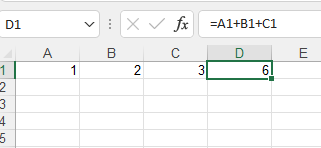python
更多相关内容
• 欢迎观看 Microsoft Excel 教程，小编带大家学习 Microsoft Excel 的使用技巧，了解如何 Excel 中创建公式并使用内置函数执行计算。

欢迎观看 Microsoft Excel 教程，小编带大家学习 Microsoft Excel 的使用技巧，了解如何在 Excel 中创建公式并使用内置函数执行计算。

Excel 中的计算方式始终以等号开始，创建引用其他单元格中的值的公式，选择单元格，键入等号「=」。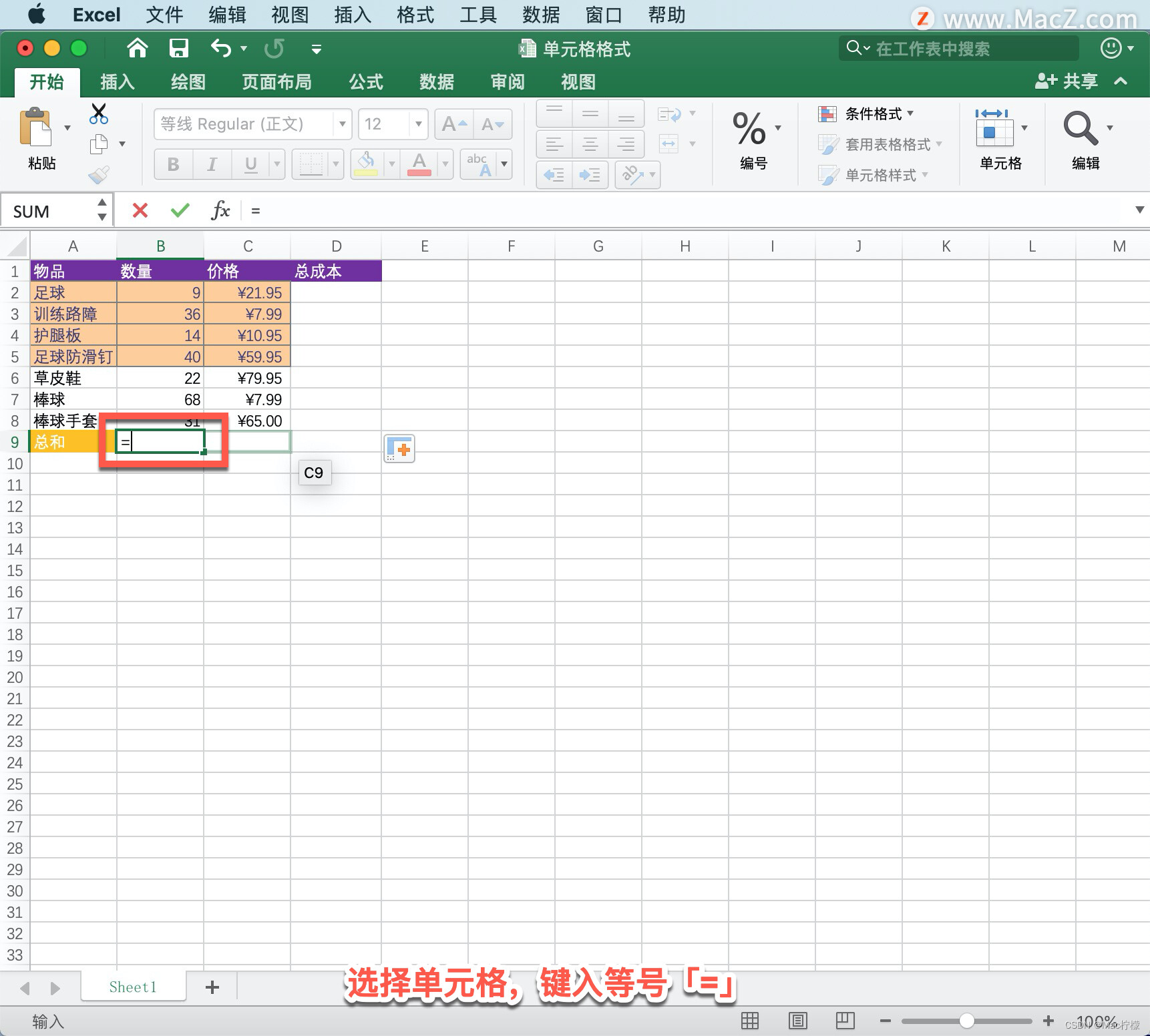Mac软件资源站 macz.com/?id=NzUxNzYwJl8mMTAxLjI3LjI1LjQ5
选择一个单元格，输入运算符，使用加号「+」添加，选择下一单元格。 例如，- 代表相减。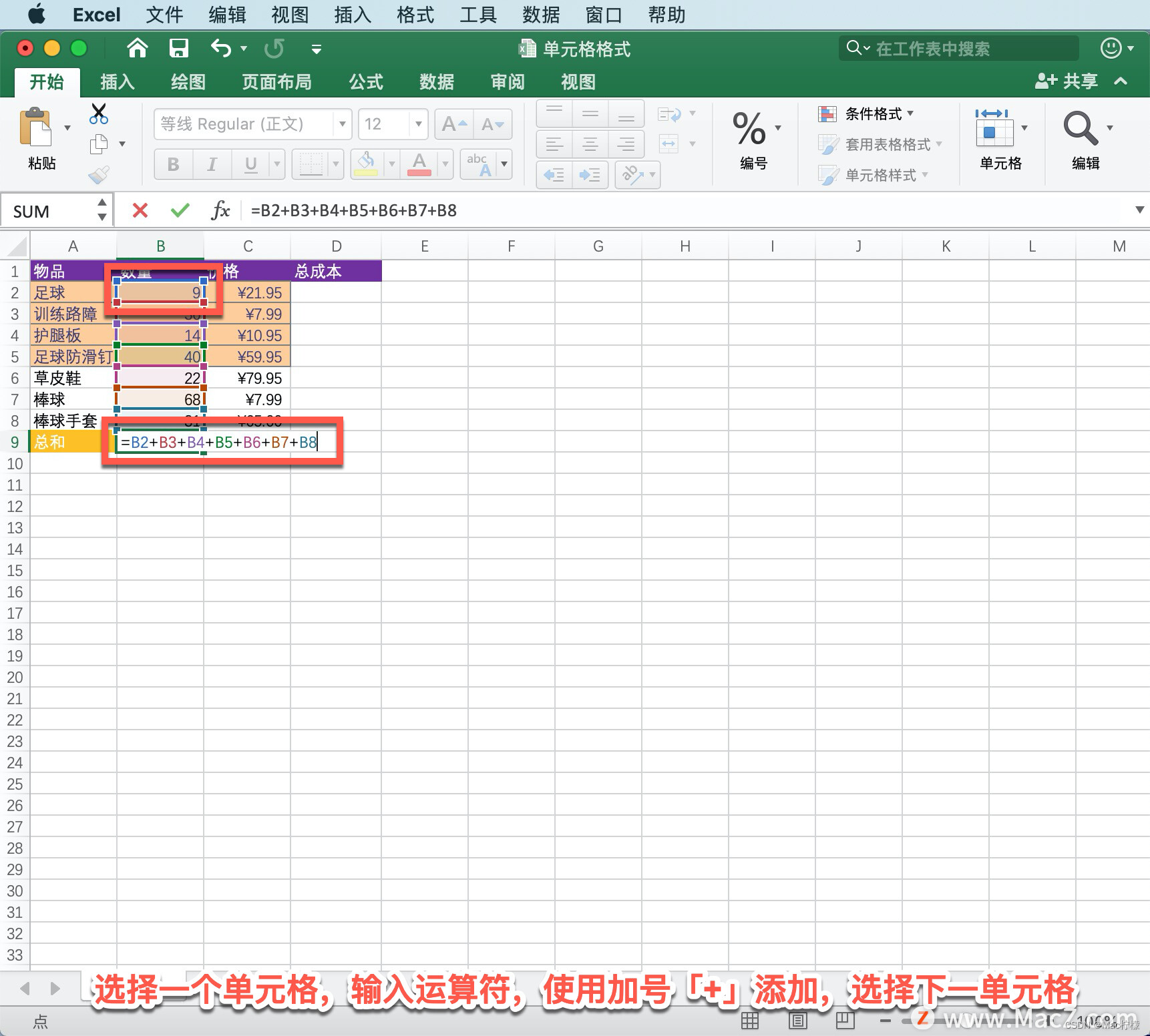按 Return 键，计算结果将显示在包含公式的单元格中。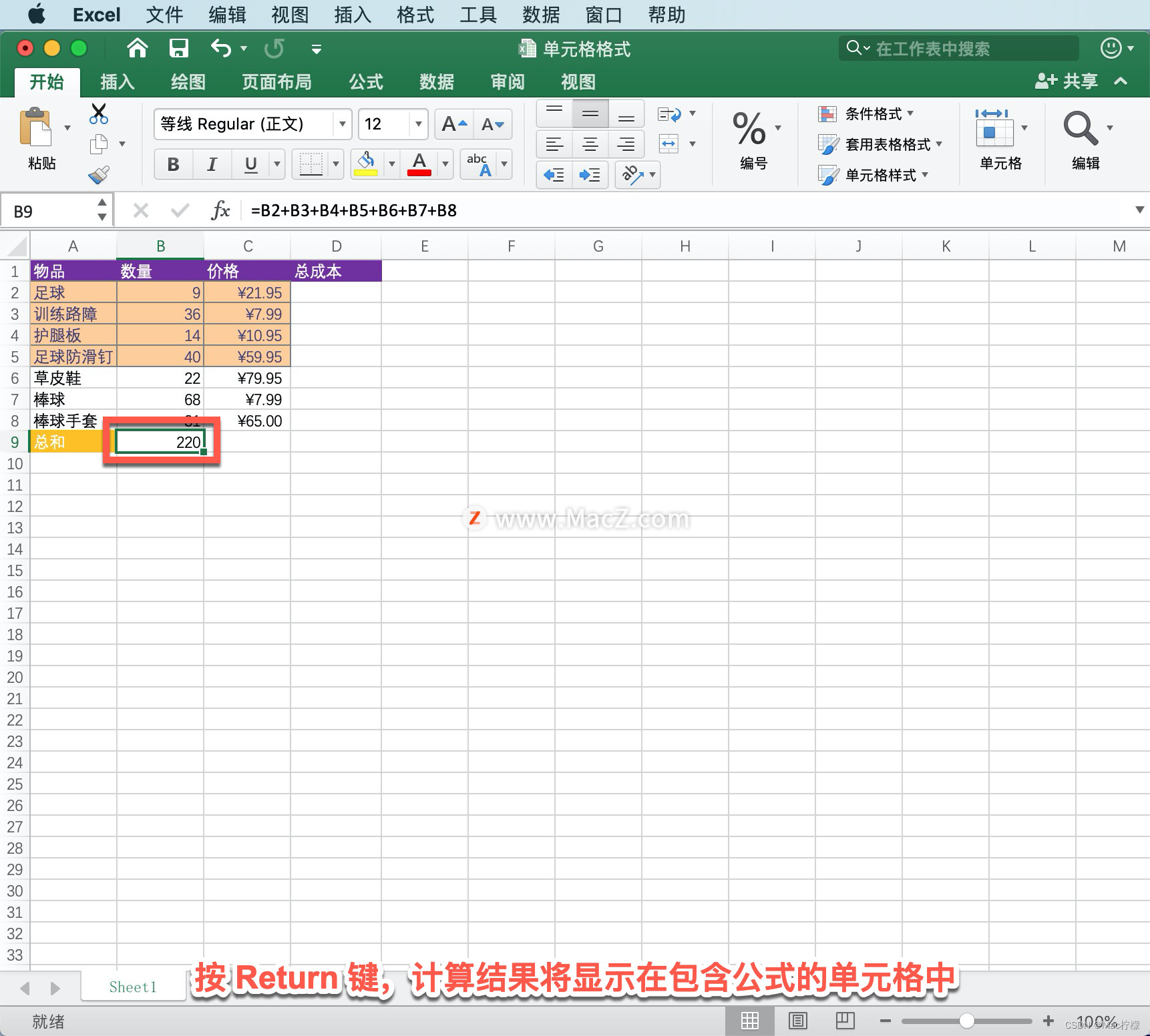在单元格中输入公式时，该公式还会出现在「编辑栏」中。

如果查看公式，选择求和的单元格，该单元格会出现在「编辑栏」中。

这是一种手动的操作方式，接下来使用一种快捷的方式函数。输入包含内置函数的公式，选择「总成本」所在的单元格，键入一个等号「=」，然后键入「SUM」函数。 例如，用「=SUM」，对添加的数字进行求和计算。

从左括号「(」开始选择单元格范围，不采用键入方式，选择要添加的第一个单元格，键入「*」并选择最后一个单元格。

按 Return 键获取结果，此时便能看到总和，但要注意上面栏中的公式，公式表示这是单元格B2乘以C2的总和。

只要设置好一个公式，即可将其复制到另一列或另一行，将光标停留在单元格的右下角，在其变成十字后进行选择并拖动。

公式会对各行进行调整，快速计算其总和。

以上就是在 Microsoft Excel 中创建公式并使用内置函数执行计算的方法。需要 Microsoft Excel 软件的朋友可在本站下载，关注本站，学习Mac软件使用方法！

展开全文• Excel中的数组是指一行、一列或多行多列的一组数据元素的集合，在Excel中用{}进行创建，其中同一行的元素用逗号“，”隔开，不同行的元素用分号“；”隔开。 如下图展示的是一个3行5列的数组： 1 2 3 4 5 6 ...
• 操作excel表格用公式来处理数据时，可通过创建公式来运算数据，或通过读取公式来获取数据信息来源。这里使用了java类库(Free Spire.XLS for Java 免费版)获取文件包后，解压，将lib文件夹下的jar文件导入Java程序。...操作excel表格用公式来处理数据时，可通过创建公式来运算数据，或通过读取公式来获取数据信息来源。这里使用了java类库(Free Spire.XLS for Java 免费版)获取文件包后，解压，将lib文件夹下的jar文件导入Java程序。

如图：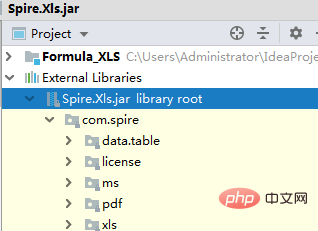(免费视频教程分享：java视频教程)

1、创建公式import com.spire.xls.*;

public class AddFormula {

public static void main(String[] args) {

//创建Workbook对象

Workbook wb = new Workbook();

//获取第一个工作表

Worksheet sheet = wb.getWorksheets().get(0);

//声明两个变量

int currentRow = 1;

String currentFormula = null;

//设置列宽

sheet.setColumnWidth(1, 32);

sheet.setColumnWidth(2, 16);

//写入用于测试的数据到单元格

sheet.getCellRange(currentRow,1).setValue("测试数据：");

sheet.getCellRange(currentRow,2).setNumberValue(1);

sheet.getCellRange(currentRow,3).setNumberValue(2);

sheet.getCellRange(currentRow,4).setNumberValue(3);

sheet.getCellRange(currentRow,5).setNumberValue(4);

sheet.getCellRange(currentRow,6).setNumberValue(5);

//写入文本

currentRow += 2;

sheet.getCellRange(currentRow,1).setValue("公式：") ; ;

sheet.getCellRange(currentRow,2).setValue("结果：");

//设置单元格格式

CellRange range = sheet.getCellRange(currentRow,1,currentRow,2);

range.getStyle().getFont().isBold(true);

range.getStyle().setKnownColor(ExcelColors.LightGreen1);

range.getStyle().setFillPattern(ExcelPatternType.Solid);

range.getStyle().getBorders().getByBordersLineType(BordersLineType.EdgeBottom).setLineStyle(LineStyleType.Medium);

//算数运算

currentFormula = "=1/2+3*4";

sheet.getCellRange(++currentRow,1).setText(currentFormula);

sheet.getCellRange(currentRow,2).setFormula(currentFormula);

//日期函数

currentFormula = "=TODAY()";

sheet.getCellRange(++currentRow,1).setText(currentFormula);

sheet.getCellRange(currentRow,2).setFormula(currentFormula);

sheet.getCellRange(currentRow,2).getStyle().setNumberFormat("YYYY/MM/DD");

//时间函数

currentFormula = "=NOW()";

sheet.getCellRange(++currentRow,1).setText(currentFormula);

sheet.getCellRange(currentRow,2).setFormula(currentFormula);

sheet.getCellRange(currentRow,2).getStyle().setNumberFormat("H:MM AM/PM");

//IF函数

currentFormula = "=IF(B1=5,\"Yes\",\"No\")";

sheet.getCellRange(++currentRow,1).setText(currentFormula);

sheet.getCellRange(currentRow,2).setFormula(currentFormula);

//PI函数

currentFormula = "=PI()";

sheet.getCellRange(++currentRow,1).setText(currentFormula);

sheet.getCellRange(currentRow,2).setFormula(currentFormula);

//三角函数

currentFormula = "=SIN(PI()/6)";

sheet.getCellRange(++currentRow,1).setText(currentFormula);

sheet.getCellRange(currentRow,2).setFormula(currentFormula);

//计数函数

currentFormula = "=Count(B1:F1)";

sheet.getCellRange(++currentRow,1).setText(currentFormula);

sheet.getCellRange(currentRow,2).setFormula(currentFormula);

//最大值函数

currentFormula = "=MAX(B1:F1)";

sheet.getCellRange(++currentRow,1).setText(currentFormula);

sheet.getCellRange(currentRow,2).setFormula(currentFormula);

//平均值函数

currentFormula = "=AVERAGE(B1:F1)";

sheet.getCellRange(++currentRow,1).setText(currentFormula);

sheet.getCellRange(currentRow,2).setFormula(currentFormula);

//求和函数

currentFormula = "=SUM(B1:F1)";

sheet.getCellRange(++currentRow,1).setText(currentFormula);

sheet.getCellRange(currentRow,2).setFormula(currentFormula);

//保存文档

wb.dispose();

}

}

公式创建结果：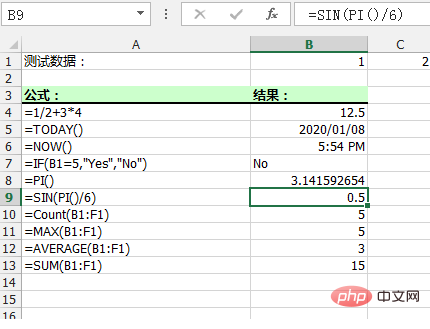2、读取公式import com.spire.xls.*;

public class ReadFormula {

public static void main(String[] args) {

//加载Excel文档

Workbook wb = new Workbook();

//获取第一个工作表

Worksheet sheet = wb.getWorksheets().get(0);

//遍历B1到B13的单元格

for (Object cell: sheet.getCellRange("B1:B13"))

{

CellRange cellRange = (CellRange)cell;

//判断单元格是否含有公式

if (cellRange.hasFormula())

{

//打印单元格及公式

String certainCell = String.format("单元格[%d, %d]含有公式：", cellRange.getRow(), cellRange.getColumn());

System.out.println(certainCell + cellRange.getFormula());

}

}

}

}

公式读取结果：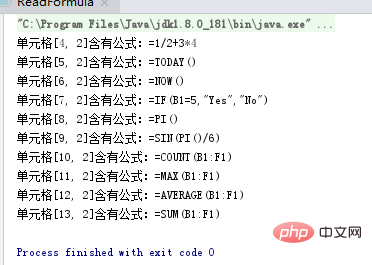相关文章教程推荐：java快速入门

展开全文• 公式解析器 库提供了一个Parser类，用于评估excel和数学公式。安装建议的安装公式分析器的方法是使用以下命令通过 ： \$ npm install hot-formula-parser --save Node.js： var FormulaParser = require ( 'hot-...
• 领悟专家技巧，释放Excel潜能　如果只是使用Excel创建简单的表格和图表，现在是时候深挖这个软件的真正功能了：公式。本书是一本理想的指南，介绍了公式的方方面面，向读者展示了Excel的强大功能。　利用本书的...
• 码农公社 210.net.cn210= 102410月24日一个重要的节日--码农(程序员)节操作excel用公式来处理数据时，可通过创建公式来运算数据，或通过读取公式来获取数据信息来源。本文以通过Java代码来演示在Excel中创建及读取...码农公社  210.net.cn  210= 1024  10月24日一个重要的节日--码农(程序员)节

操作excel用公式来处理数据时，可通过创建公式来运算数据，或通过读取公式来获取数据信息来源。

本文以通过Java代码来演示在Excel中创建及读取公式的方法。

这里使用了Excel Java类库(Free Spire.XLS for Java 免费版)，在官网下载获取文件包后，

解压，将lib文件夹下的jar文件导入Java程序；或者通过maven仓库下载并导入。

导入结果如下：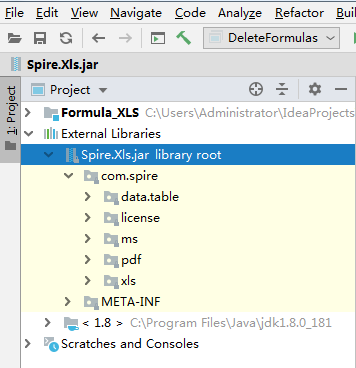1.创建公式

import com.spire.xls.*;

public class AddFormula {

public static void main(String[] args) {

//创建Workbook对象

Workbook wb = new Workbook();

//获取第一个工作表

Worksheet sheet = wb.getWorksheets().get(0);

//声明两个变量

int currentRow = 1;

String currentFormula = null;

//设置列宽

sheet.setColumnWidth(1, 32);

sheet.setColumnWidth(2, 16);

//写入用于测试的数据到单元格

sheet.getCellRange(currentRow,1).setValue("测试数据：");

sheet.getCellRange(currentRow,2).setNumberValue(1);

sheet.getCellRange(currentRow,3).setNumberValue(2);

sheet.getCellRange(currentRow,4).setNumberValue(3);

sheet.getCellRange(currentRow,5).setNumberValue(4);

sheet.getCellRange(currentRow,6).setNumberValue(5);

//写入文本

currentRow += 2;

sheet.getCellRange(currentRow,1).setValue("公式：") ; ;

sheet.getCellRange(currentRow,2).setValue("结果：");

//设置单元格格式

CellRange range = sheet.getCellRange(currentRow,1,currentRow,2);

range.getStyle().getFont().isBold(true);

range.getStyle().setKnownColor(ExcelColors.LightGreen1);

range.getStyle().setFillPattern(ExcelPatternType.Solid);

range.getStyle().getBorders().getByBordersLineType(BordersLineType.EdgeBottom).setLineStyle(LineStyleType.Medium);

//算数运算

currentFormula = "=1/2+3*4";

sheet.getCellRange(++currentRow,1).setText(currentFormula);

sheet.getCellRange(currentRow,2).setFormula(currentFormula);

//日期函数

currentFormula = "=TODAY()";

sheet.getCellRange(++currentRow,1).setText(currentFormula);

sheet.getCellRange(currentRow,2).setFormula(currentFormula);

sheet.getCellRange(currentRow,2).getStyle().setNumberFormat("YYYY/MM/DD");

//时间函数

currentFormula = "=NOW()";

sheet.getCellRange(++currentRow,1).setText(currentFormula);

sheet.getCellRange(currentRow,2).setFormula(currentFormula);

sheet.getCellRange(currentRow,2).getStyle().setNumberFormat("H:MM AM/PM");

//IF函数

currentFormula = "=IF(B1=5,\"Yes\",\"No\")";

sheet.getCellRange(++currentRow,1).setText(currentFormula);

sheet.getCellRange(currentRow,2).setFormula(currentFormula);

//PI函数

currentFormula = "=PI()";

sheet.getCellRange(++currentRow,1).setText(currentFormula);

sheet.getCellRange(currentRow,2).setFormula(currentFormula);

//三角函数

currentFormula = "=SIN(PI()/6)";

sheet.getCellRange(++currentRow,1).setText(currentFormula);

sheet.getCellRange(currentRow,2).setFormula(currentFormula);

//计数函数

currentFormula = "=Count(B1:F1)";

sheet.getCellRange(++currentRow,1).setText(currentFormula);

sheet.getCellRange(currentRow,2).setFormula(currentFormula);

//最大值函数

currentFormula = "=MAX(B1:F1)";

sheet.getCellRange(++currentRow,1).setText(currentFormula);

sheet.getCellRange(currentRow,2).setFormula(currentFormula);

//平均值函数

currentFormula = "=AVERAGE(B1:F1)";

sheet.getCellRange(++currentRow,1).setText(currentFormula);

sheet.getCellRange(currentRow,2).setFormula(currentFormula);

//求和函数

currentFormula = "=SUM(B1:F1)";

sheet.getCellRange(++currentRow,1).setText(currentFormula);

sheet.getCellRange(currentRow,2).setFormula(currentFormula);

//保存文档

wb.dispose();

}

}

公式创建结果：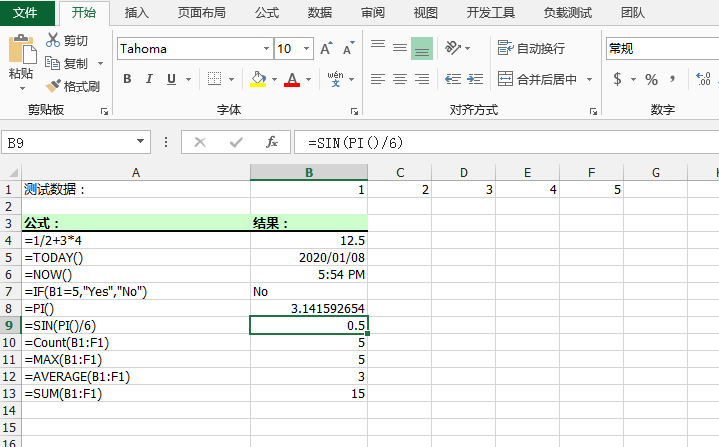2.读取公式

import com.spire.xls.*;

public class ReadFormula {

public static void main(String[] args) {

//加载Excel文档

Workbook wb = new Workbook();

//获取第一个工作表

Worksheet sheet = wb.getWorksheets().get(0);

//遍历B1到B13的单元格

for (Object cell: sheet.getCellRange("B1:B13"))

{

CellRange cellRange = (CellRange)cell;

//判断单元格是否含有公式

if (cellRange.hasFormula())

{

//打印单元格及公式

String certainCell = String.format("单元格[%d, %d]含有公式：", cellRange.getRow(), cellRange.getColumn());

System.out.println(certainCell + cellRange.getFormula());

}

}

}

}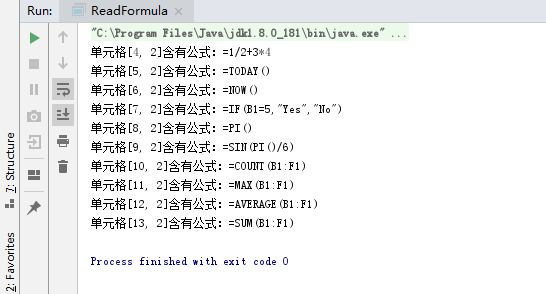展开全文• 1.3 在公式中使用函数 14 1.3.1 为什么使用函数 14 1.3.2 函数的类型 14 1.3.3 函数的参数 15 1.3.4 在公式中输入函数 16 1.4 在公式中使用名称 18 1.4.1 名称的作用范围 19 1.4.2 命名区域 19 1.4.3 命名...
• How can I construct the following string in an Excel formula: 如何在Excel公式中构造以下字符串： Maurice "The
• excel表格的乘法公式怎么用1、首先在Excel表格输入几组需要计算的数据，需要将数据相乘后再相加。2接E1单中输入公式：=A1*B1 C1*D1。3、点击回车即可得到计算结果，因为乘法公式先于加法公式，所以不需要添加...
• 领悟专家技巧，释放Excel潜能　如果只是使用Excel创建简单的表格和图表，现在是时候深挖这个软件的真正功能了：公式。本书是一本理想的指南，介绍了公式的方方面面，向读者展示了Excel的强大功能。　利用本书的...
• excel创建下拉列表多选Excel provides the drop-down list in order to provide multiple options to select one. The drop-down list can be created in different ways and options. In this tutorial, we will ...java python
• 常量：直接输入到公式中的数字或文本值，例如 2。 运算符：^（脱字号）运算符表示数字的乘方，而 *（星号）运算符表示数字的乘积。 引用规则 对名为 Marketing 的工作表的引用 引用 B1 到 B10 的单元格区域 感叹...
• excel公式失效Say you’ve created an Excel workbook you need to distribute, but you can’t reveal the formulas you used in that workbook. We’ll show you an easy trick that allows you to copy a ...java
• 原文：http://coolketang.com/staticOffice/5a97f34f9f545442015d39ed.html1. 本节课将为您演示，如何插入图形，并图形输入计算公式。首先通过公式获得成绩表总分最高的学生姓名。点击选择J3单元格。 2. ...
• 如何在excel中创建“数据库”Excel创建方法1、首先新建一个Excel2、新建 Microsoft Excel 工作表要编辑的内容。如图：3、所编辑的内容，名称框输入数据库的名称并按回车键。(注：此把新建的数据库命名为...mysql的表在哪里
• Your Excel data changes frequently, so it’s useful to create a dynamic defined range that automatically expands and contracts to the size of your ... Excel数据经常更改，因此创建动态定义的范围会自动...python java 大数据
• 第1章Excel2010的基本操作、第二章函数和公式的基础知识、第三章财务类函数的应用、第四章逻辑类函数的应用、第五章文本类函数的应用、第六章日期与时间函数的应用、第七章查找与引用函数的应用、第八章数学和三角...
• 本文，我将向您展示如何使用Free Spire.XLS for Java在Excel单元格应用公式和函数。 Add Spire.Xls.jar as dependency Method 1: Download Free Spire.XLS for Java pack, unzip it and you’ll get Spire....java
• Excel 创建公式实现 选中单元格查询满足条件的值，返回第二列的内容拼接为数组
• excel中计算表格中公式 在Excel中计算年度成本和节省 (Calculate Annual Costs and Savings in Excel) This workbook shows how to calculate annual costs and savings in Excel. It’s amazing how all those ...
• 如果只是使用Excel创建简单的表格和图表，现在是时候深挖这个软件的真正功能了：公式。本书是一本理想的指南，介绍了公式的方方面面，向读者展示了Excel的强大功能。 利用本书的提示和技巧，读者的工作流程将变得...
• 如何通过Java代码实现创建和读取Excel公式发布时间：2020-06-06 21:58:24来源：亿速云阅读：250作者：Leah如何通过Java代码实现创建和读取Excel公式？相信大部分人都还没学会这个技能，为了让大家学会，给大家总结了...
• 然后它创建一个新的 Excel 文件，其中包含 2N+1 个工作表（其中 N 是原始工作簿的工作表数）。 每个奇数工作表 2n-1 按行顺序列出工作表 n（来自原始文件）的单元格名称、值和公式，每张工作表 2n 按列顺序列出...matlab
• 您可能感兴趣的文章:Python读取excel文件公式的值的实现利用python在excel中画图的实现方法python读写Excel表格的实例代码（简单实用）使用 Python 合并多个格式一致的 Excel 文件(推荐)Python使用Pandas读写...
• 所以我知道这是一篇很老的文章，但是我找到了一个很好的方法，可以从工作簿的所有工作表获取公式，也可以让新创建的工作簿保留所有格式。第一步是将.xlsx文件的副本保存为.xls--下面的代码使用.xls作为...
• 通过Excel管理表结构自动生成创建表sql文支持（sqlserver，oracle）
• Excel 工作表如何快速的...如果一个一个的输入的话，不仅麻烦而且要花费很长时间，但是 Excel 有一些的小技巧可以帮助我们快速的应用一个公式到一整列。Recommended Productivity Software Use tabbed in......